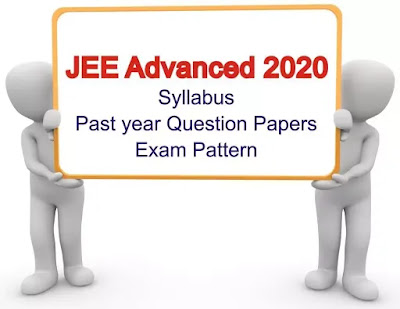# JEE Advanced 2020 paper 1 & 2 syllabus

## JEE Advanced 2020 syllabus, exam pattern, past Year question papers for Physics, Chemistry, Maths, and Architecture

IIT Delhi is a conducting authority for this year to conduct JEEAdvanced in 2020. IIT Delhi has been released a notification for JEE Advanced 2020 paper 1 & 2 syllabus at the official website. Candidates have must know the JEE Advanced 2020 syllabus for better performance in JEE Advanced examination. The Syllabus of Jee advance is very helpful the candidates who are preparing for this examination.JEE Advanced 2020

But Maybe the syllabus will be reduced by the respective authorities for JEE Advanced 2020. Due to the rising of COVID 19 pandemic issue, required to maintain social distancing and other safety reasons. Maybe the final syllabus for the jee advance will be announced in this week (July 15, 2020) as per the comment of IIT director, V. Ramgopal Rao.

As per the current details for JEE Advanced 2020 paper 1 & 2, the syllabus consists of the section-wise Physics, Chemistry, Mathematics and Architecture Aptitude Tes (AAT). Candidates can check the syllabus along with the exam pattern and reference books below for the reference. Candidates download subject wise PDF file for the full syllabus details.

### JEE Advanced 2020 Syllabus for Maths

 Algebra Algebra of complex numbers, addition, multiplication, conjugation, polar representation, properties of modulus and principal argument, triangle inequality, cube roots of unity, geometric interpretations. Matrices Matrices as a rectangular array of real numbers, equality of matrices, addition, multiplication by a scalar and product of matrices, transpose of a matrix, determinant of a square matrix of order up to three, inverse of a square matrix of order up to three, properties of these matrix operations, diagonal, symmetric and skew-symmetric matrices and their properties, solutions of simultaneous linear equations in two or three variables. Probability Addition and multiplication rules of probability, conditional probability, Bayes Theorem, independence of events, computation of probability of events using permutations and combinations. Trigonometry Trigonometric functions, their periodicity and graphs, addition and subtraction formulae, formulae involving multiple and sub-multiple angles, general solution of trigonometric equations. Analytical geometry dimensions: Cartesian coordinates, distance between two points, section formulae, shift of origin. Differential calculus Real valued functions of a real variable, into, onto and one-to-one functions, sum, difference, product and quotient of two functions, composite functions, absolute value, polynomial, rational, trigonometric, exponential and logarithmic functions. Integral calculus Integration as the inverse process of differentiation, indefinite integrals of standard functions, definite integrals and their properties, fundamental theorem of integral calculus. Vectors Addition of vectors, scalar multiplication, dot and cross products, scalar triple products and their geometrical interpretations.

### JEE Advanced 2020 Syllabus for Architecture

 Freehand drawing This would comprise of simple drawing depicting the total object in its right form and proportion, surface texture, relative location and details of its component parts in appropriate scale. Common domestic or day-to-day life usable objects like furniture, equipment, etc., from memory. Geometrical drawing Exercises in geometrical drawing containing lines, angles, triangles, quadrilaterals, polygons, circles, etc. Study of plan (top view), elevation (front or side views) of simple solid objects like prisms, cones, cylinders, cubes, splayed surface holders, etc. Three-dimensional perception Understanding and appreciation of three-dimensional forms with building elements, colour, volume and orientation. Visualization through structuring objects in memory. Imagination and aesthetic sensitivity Composition exercise with given elements. Context mapping. Creativity check through innovative uncommon test with familiar objects. Sense of colour grouping or application. Architectural awareness General interest and awareness of famous architectural creations – both national and international, places and personalities (architects, designers, etc.) in the related domain.

### JEE Advanced Previous Year Paper

All the Question papers source from the official website.

### Section 1: Single Correct Option type questions

Section 2:One or more option (s) is correct type questions and

### Section 1: One or more option (s) is the correct type questions

Section 2: Numerical Value type question and